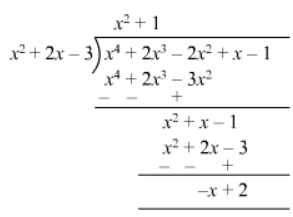# What must be added to`
Question:

What must be added to x4 + 2x3 − 2x2 + x − 1 , so that the resulting polynomial is exactly divisible by x2 + 2x − 3?

Solution:Thus, $(x-2)$ should be added to $\left(x^{4}+2 x^{3}-2 x^{2}+x-1\right)$ to make the resulting polynomial exactly divisible by $\left(x^{2}+2 x-3\right)$